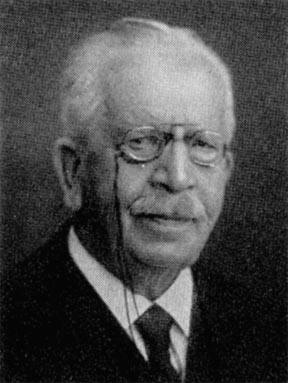# Friedrich Wilhelm August Ludwig Kiepert (1846-1934) mathematician

 One of the best known conic sections in triangle geometry is the Kiepert hyperbola, named after Ludwig Kiepert. Suppose ABC is a triangle. Let A' be a point free to move along the perpendicular bisector, L, of side BC. Let B' be the point on the perpendicular bisector of side CA for which triangle CB'A is similar to triangle BA'C, and let C' be the point on the perpendicular bisector of side AB for which triangle AC'B is similar to triangle CB'A. The three lines AA', BB', CC' concur in a point, K. As A' traces L, point K traces the Kiepert hyperbola of triangle ABC. Kiepert was professor of mathematics at Hannover Technische Hochschule from 1879 to 1921 and rector from 1901 to 1904. His Ph.D. was taken at the University of Berlin in 1870 under Karl Weierstrass.He wrote a calculus textbook in 1863, Grundriss der Integral-Rechnung. A measure of the success of this book is that it ran through fourteen editions. Published in two volumes, the second was essentially a textbook on ordinary differential equations. Kiepert's research publications are listed in Poggendorff's Handbuch, volumes 3-5.

Graphs of the Kiepert hyperbola and Kiepert parabola, a discussion of much related geometry, and accounts of rediscoveries of the Kiepert hyperbola are given in

R. H. Eddy and R. Fritsch, "The Conics of Ludwig Kiepert: A Comprehensive Lesson in the Geometry of the Triangle," Mathematics Magazine 67 (1994) 188-205.